# OpenGL学习脚印: 向量和矩阵要点(math-vector and matrices)

• 向量基本概念和操作
• 矩阵的基本概念和操作
• GLM数学库

## 向量的概念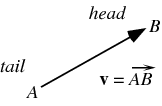1.向量的大小就是向量的长度（模）。向量的长度非负。
2.向量的方向描述了向量的指向。
3.向量是没有位置的，与点是不同的。
4.向量与标量不同，变量是只有大小而没有方向的量，例如位移是向量，而距离是标量。

## 零向量与单位向量

|v|=v21+v22++v2n$|v|=\sqrt{v_{1}^2 + v_{2}^2+\cdots+v_{n}^2}$
|v|=ni=1v2i$|v|=\sqrt{\sum_{i=1}^{n}v_{i}^2}$

vnorm=v|v|,v0$v_{norm}=\frac{v}{| v|},v \neq 0$

## 三角形法则和平行四边形法则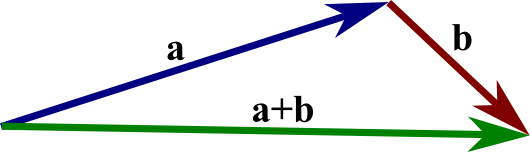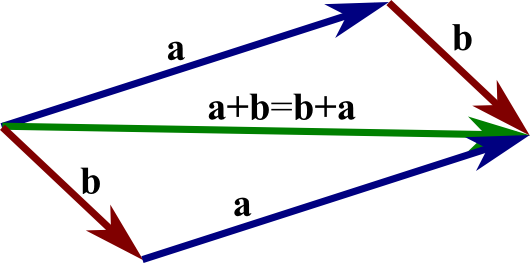a+(a)=0$a+(-a)=0$

## 向量夹角

0θπ$0 \leq \theta \leq \pi$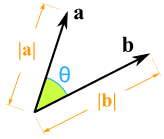## 向量点积(dot product)

A.B=|A||B|cosθ(1)$A.B=|A||B|cos\theta\tag{1}$

AB=|A|cosθ(2)$A_{B}=|A|cos\theta\tag{2}$A.B=|A|BA=|B|AB(3)$A.B = |A|B_{A} = |B|A_{B}\tag{3}$

A=(a1,b2,,cn)$A=(a_{1}, b_{2},\cdots,c_{n})$B=(b1,b2,,bn)$B=(b_{1},b_{2},\cdots, b_{n})$,

A.B=a1b1+a2b2++anbn=i=1naibi(4)

cosθ=a.b|a||b|(5)$cos\theta = \frac{a.b}{|a||b|}\tag{5}$

cosθ=a.b(6)$cos\theta = a.b\tag{6}$

|a|2=aa(7)$|a|^{2}=a \cdot a \tag{7}$

## 向量的叉积(cross product)

|c|=|a||b|sinθ(8)$|c|=|a||b|sin\theta\tag{8}$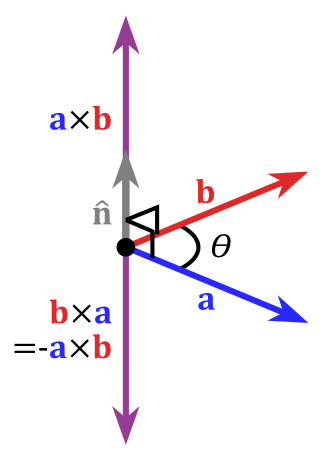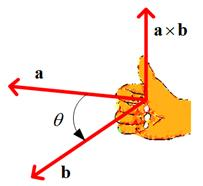a×b=b×a(9)$a \times b = - b \times a\tag{9}$

c=a×b=iaxbxjaybykazbz=[aybyazbz]i[axbxazbz]j+[axbxayby]k=aybzazbyazbxaxbzaxbyaybx(10)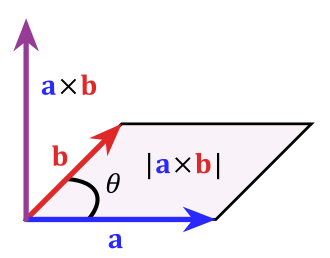## 投影向量的计算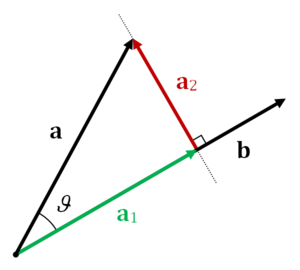a1=|a1|b|b|=|a|cosθb|b|=(|a||b|cosθ)b|b|2=ab|b|2b(11)

a2=aa1=aab|b|2b(12)$a_{2}=a - a_{1}=a- \frac{a \cdot b}{|b|^{2}}b \tag{12}$

## 矩阵的概念

147258369$\begin{bmatrix} 1 & 2 & 3 \\ 4 & 5 & 6 \\ 7 & 8 & 9\end{bmatrix}$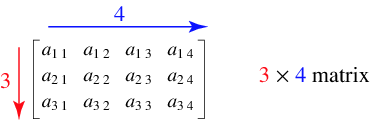## 行向量和列向量

a=axayaz$a=\begin{bmatrix} a_{x} \\ a_{y} \\ a_{z}\end{bmatrix}$

## 零矩阵和n阶单位阵

mxn矩阵，如果所有元素都为0，则成为零矩阵。

A=

AT=123456.

## 矩阵的运算

### 矩阵加减法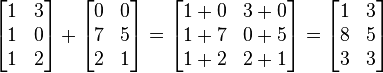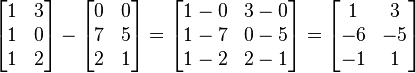### 标量和矩阵乘法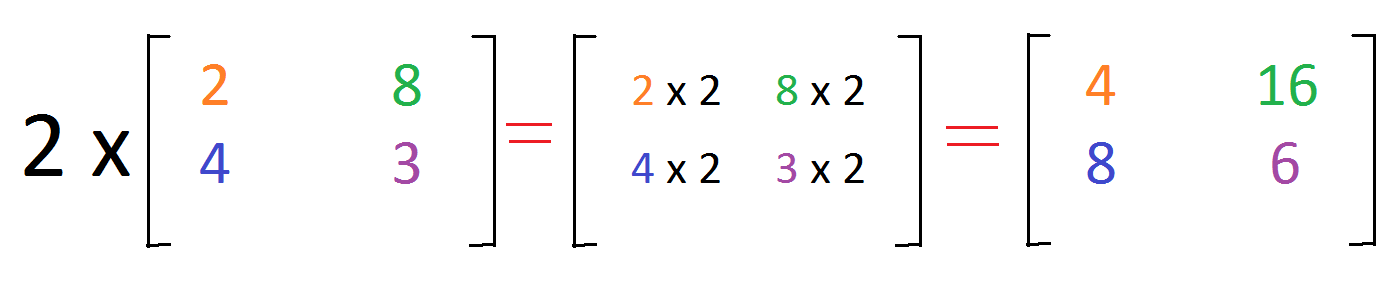### 矩阵和矩阵乘法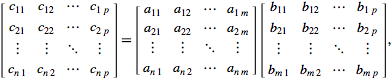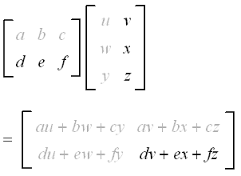#### 矩阵和矩阵相乘举例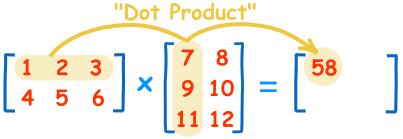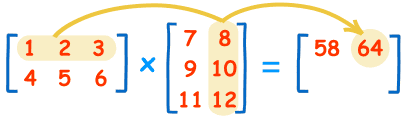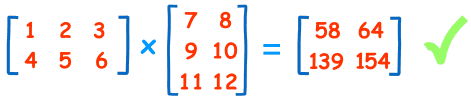a=(a1,b2,,cn)$a=(a_{1}, b_{2},\cdots,c_{n})$b=(b1,b2,,bn)$b=(b_{1},b_{2},\cdots, b_{n})$,

a.b=a1b1+a2b2++anbn=i=1naibi=[a1a2an]b1b2bn=aTb(13)

#### 矩阵不满足交换律举例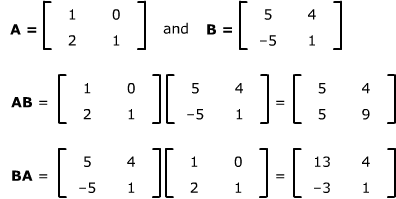### 矩阵和向量相乘

Av=147102581136912210=21+12+0324+15+0627+18+09210+111+012=0369.

## 行列式

A=[acbd]$A=\begin{bmatrix} a & b \\ c & d \end{bmatrix}$

det(A)=acbd=adbc

3x3矩阵的行列式计算如下:
detadgbehcfi=adet([ehfi])bdet([dgfi])+cdet([dgeh])=a(eifh)b(difg)+c(dheg)=aei+bfg+cdhafhbdiceg

## 逆矩阵

AB=BA=I(14)$AB=BA=I \tag{14}$

I3x3=100010001$I_{3x3}=\begin{bmatrix} 1 & 0 & 0 \\ 0 & 1 & 0 \\ 0 & 0 & 1\end{bmatrix}$

n阶方阵A可逆的充要条件是A的行列式|A|0$|A| \neq 0$.

M1(Mv)=(M1M)v=Iv=v$M^{-1}(Mv)=(M^{-1}M)v=Iv=v$

## 正交矩阵

MMMT=IMT=M1(15)$M正交\Leftrightarrow MM^{T}=I \Leftrightarrow M^{T}=M^{-1}\tag{15}$

An×nβiβTj={1,0,i=jij(16)$A_{n \times n}正交 \Leftrightarrow \beta_{i}\beta_{j}^{T}= \begin{cases} 1, & i=j \\ 0, & i \neq j \end{cases} \tag{16}$

• 矩阵的每一行都是单位向量
• 矩阵的所有行互相垂直。

### 正交矩阵举例

Rx(θ)=10000cosθsinθ00sinθcosθ00001$R_{x}(\theta)=\begin{bmatrix} 1 & 0 & 0 & 0 \\ 0 & cos\theta & -sin\theta & 0 \\ 0 & sin\theta & cos\theta & 0 \\ 0 & 0 & 0 & 1\end{bmatrix}$

Rx(θ)1=Rx(θ)(*)$R_{x}(\theta)^{-1}=R_{x}(-\theta) \tag{*}$

Rx(θ)=10000cosθsinθ00sinθcosθ00001$R_{x}(-\theta)=\begin{bmatrix} 1 & 0 & 0 & 0 \\ 0 & cos\theta & sin\theta & 0 \\ 0 & -sin\theta & cos\theta & 0 \\ 0 & 0 & 0 & 1\end{bmatrix}$

Rx(θ)=Rx(θ)T(**)$R_{x}(-\theta)=R_{x}(\theta)^{T} \tag{**}$

Rx(θ)1=Rx(θ)T(***)$R_{x}(\theta)^{-1}=R_{x}(\theta)^{T} \tag{***}$

## GLM数学库中的向量和矩阵

GLM是一个C++编写的，基于OpenGL着色器语言规范编写只是用头文件的图形开发数学库。这个库中提供了我们需要的很多数学操作，例如包含本节提到的向量和矩阵。例如下面的代码是用了向量的标准化、叉积等操作求取了一个三角形的法向量:

    #include <glm/vec3.hpp>// glm::vec3
#include <glm/geometric.hpp>// glm::cross, glm::normalize
void computeNormal(triangle & Triangle)
{
glm::vec3 const & a = Triangle.Position;
glm::vec3 const & b = Triangle.Position;
glm::vec3 const & c = Triangle.Position;
Triangle.Normal = glm::normalize(glm::cross(c - a, b - a));
} 

## 参考资料

1.《3D数学基础：图形与游戏开发》清华大学出版社
2.《线性代数》武汉大学数学与统计学院 高等教育出版社 齐民友主编
3. 《交互式计算机图形学-基于OpenGL着色器的自动向下方法》电子工业出版社 Edward Angle等著